# OpenCV3入门（七）图像形态学

2019/04/10 10:10

## 1、膨胀

A⊕S={z|(S)z ∩ A ≠ Ø}

``````CV_EXPORTS_W void dilate( InputArray src, OutputArray dst, InputArray kernel,
Point anchor = Point(-1,-1), int iterations = 1,
int borderType = BORDER_CONSTANT,
const Scalar& borderValue = morphologyDefaultBorderValue() );``````

dst表示处理的结果

src表示原图像

kernel表示卷积核

anchor是point类型，表示锚的位置，默认正中心

iterations表示迭代次数

borderType边缘的模糊方式

CV_EXPORTS_W Mat getStructuringElement(int shape, Size ksize, Point anchor = Point(-1,-1));

MORPH_RECT：矩形

MORPH_CROSS：交叉型

MORPH_ELLIPSE：椭圆型

``````img = imread("D:\\WORK\\5.OpenCV\\LeanOpenCV\\pic_src\\pic7.bmp");

img1 = img.clone();
imshow("原图", img);
Mat element = getStructuringElement(MORPH_RECT, Size(5, 5));
dilate(img, img2, element);
imshow("膨胀", img2);``````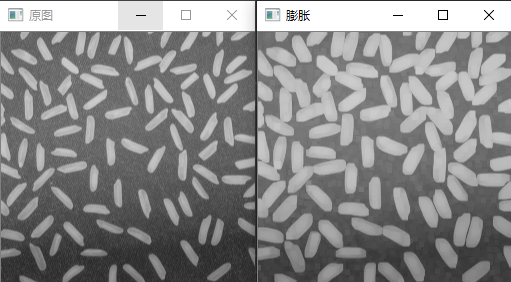## 2、腐蚀

AΘS={z|(S)z € A}

``````CV_EXPORTS_W void erode( InputArray src, OutputArray dst, InputArray kernel,
Point anchor = Point(-1,-1), int iterations = 1,
int borderType = BORDER_CONSTANT,
const Scalar& borderValue = morphologyDefaultBorderValue() );``````

``````img = imread("D:\\WORK\\5.OpenCV\\LeanOpenCV\\pic_src\\pic7.bmp");

img1 = img.clone();
imshow("原图", img);
Mat element = getStructuringElement(MORPH_RECT, Size(5, 5));
erode(img, img2, element);
imshow("腐蚀", img2);``````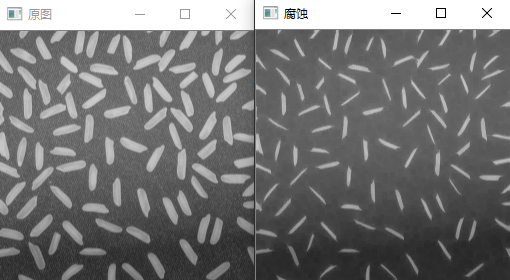## 3、开运算和闭运算

1）开运算就是先腐蚀后膨胀的过程，可以表示为：

dst = open(src, element)

dst = dilate(erode(dst, element))

2）闭运算是先膨胀后腐蚀的过程，可以表示为：

dst = close(src, element)

dst = erode(dilate (dst, element))

``````CV_EXPORTS_W void morphologyEx( InputArray src, OutputArray dst,
int op, InputArray kernel,
Point anchor = Point(-1,-1), int iterations = 1,
int borderType = BORDER_CONSTANT,
const Scalar& borderValue = morphologyDefaultBorderValue() );``````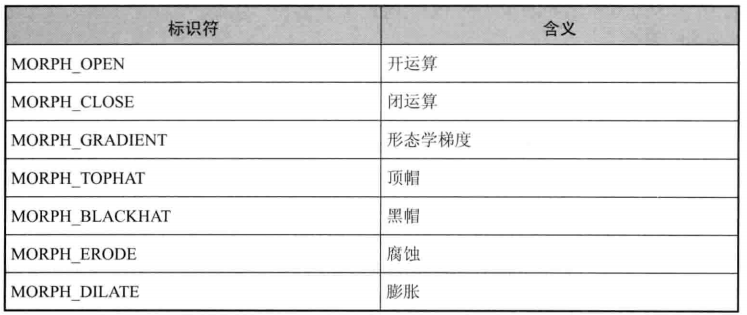``````img = imread("D:\\WORK\\5.OpenCV\\LeanOpenCV\\pic_src\\pic7.bmp");

img1 = img.clone();
imshow("原图", img);
Mat element = getStructuringElement(MORPH_RECT, Size(7, 7));
morphologyEx(img, img2, MORPH_OPEN, element);
imshow("开运算", img2);
morphologyEx(img, img3, MORPH_CLOSE, element);
imshow("闭运算", img3);``````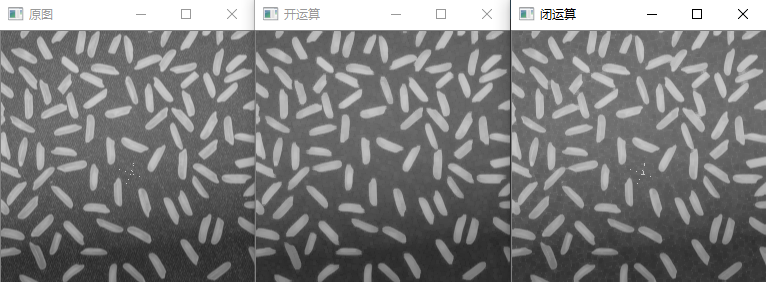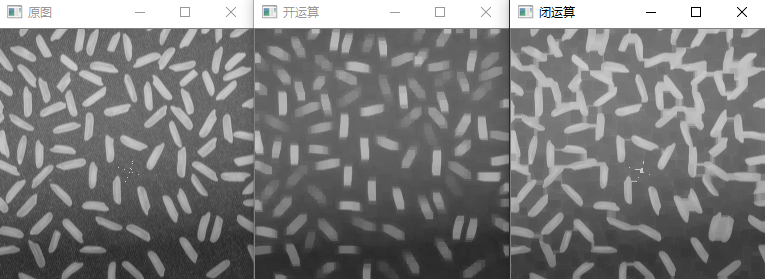## 4、形态学梯度

dst = morpd_grad(src, element) = dilate (src, element) - erode(src, element)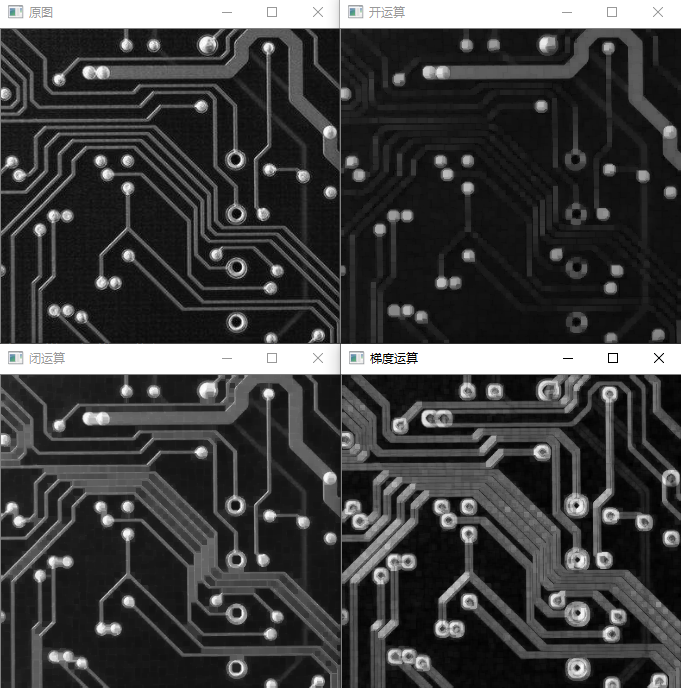## 5、顶帽和黑帽

1）顶帽（Top Hat）：

2）黑帽（Black Hat）：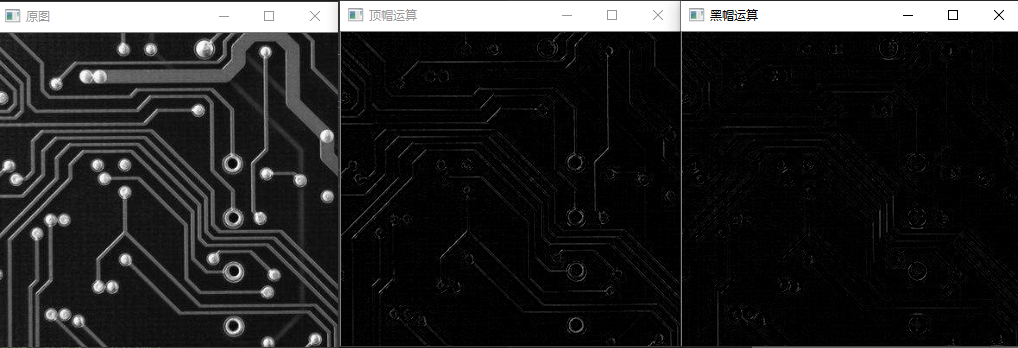## 6、高斯金字塔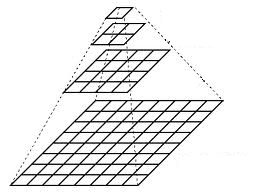1）下采样：就是图片缩小，使用PryDown函数，先高斯模糊，再降采样。下采样将步骤：先对图像进行高斯内核卷积，再将所有偶数行和列去除，图像缩小到原来四分之一，迭代上述步骤就得到的金字塔。

2）上采样：就是图片放大，使用PryUp函数。先将图像在每个方向扩大为原来的两倍，新增的行和列以0填充，再卷积得到放大后的图像。

``````CV_EXPORTS_W void pyrUp( InputArray src, OutputArray dst,
const Size& dstsize = Size(), int borderType = BORDER_DEFAULT );
CV_EXPORTS_W void pyrDown( InputArray src, OutputArray dst,
const Size& dstsize = Size(), int borderType = BORDER_DEFAULT );``````

``````img = imread("D:\\WORK\\5.OpenCV\\LeanOpenCV\\pic_src\\pic5.bmp");
imshow("原图", img);
pyrDown(img, img2, Size(img.cols * 2, img.rows * 2));
imshow("上采样运算1", img2);
pyrDown(img2, img3, Size(img2.cols * 2, img2.rows * 2));
imshow("上采样运算2", img3);``````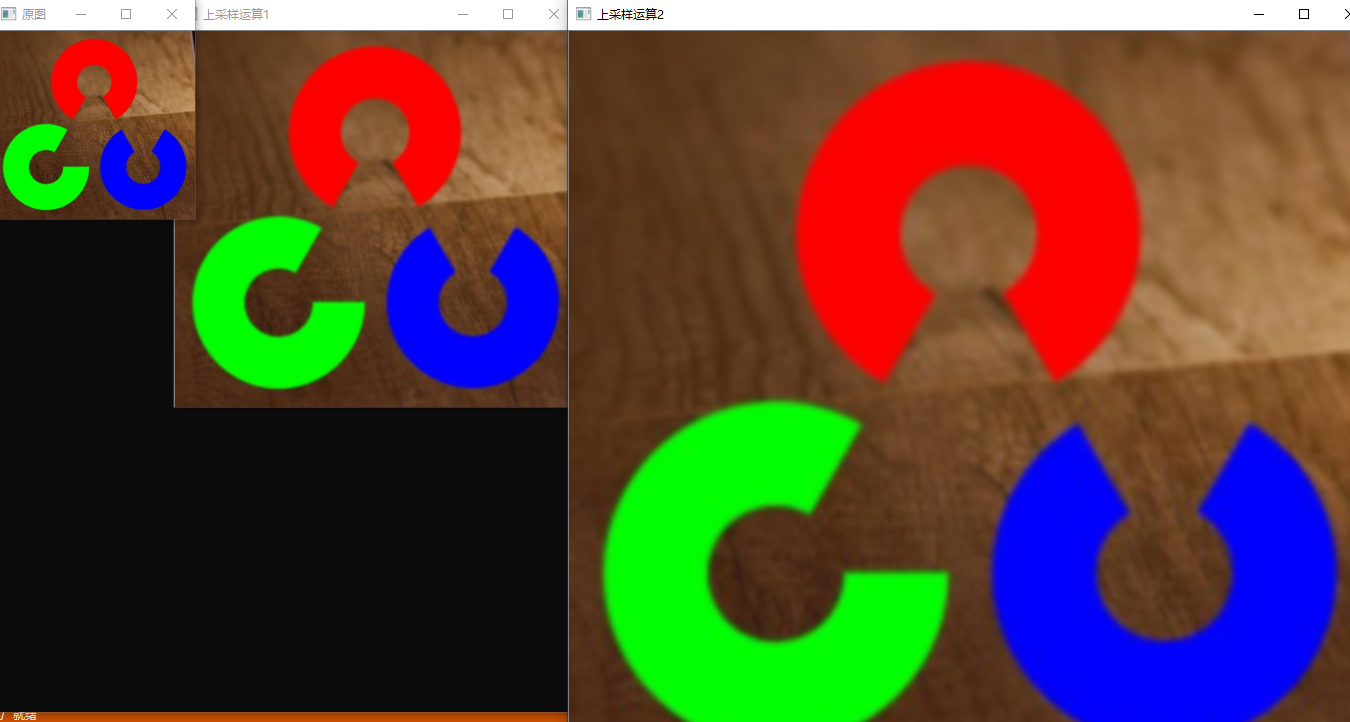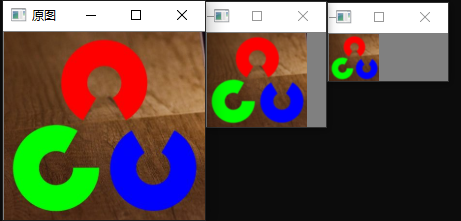## 7、参考文献

1、《OpenCV3 编程入门》，电子工业出版社，毛星雨著

3、图像金字塔（高斯金字塔、拉普拉斯金字塔）

https://www.cnblogs.com/sddai/p/10330756.html

4、图像金字塔

https://www.kancloud.cn/aollo/aolloopencv/272073

5、Image Pyramids

6、Image Pyramids

https://docs.opencv.org/2.4/doc/tutorials/imgproc/pyramids/pyramids.html

尊重原创技术文章，转载请注明。

https://www.cnblogs.com/pingwen/p/12319629.html

0
0 收藏

### 作者的其它热门文章0 评论
0 收藏
0